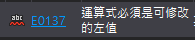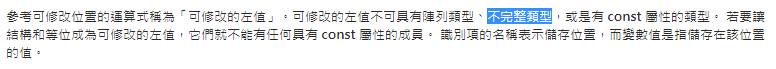#2019 iT 邦幫忙鐵人賽DAY 7
1

## Days 7: 重溫指標：數據、數據類型的本質

#### ▌前言提到：

`function(int **get_a)` 可以換成 `function(int *get_a)`

`function(void *get_a)` 呢？ （int* -> void*）

#### ▌數據本質：

sizeof(int) = 4
sizeof(double) = 8;

sizeof(void*) = 4;
sizeof(int*) = 4;
sizeof(long*) = 4;
sizeof(float*) = 4;
sizeof(double*) = 4;
sizeof(結構體*) = 4;
sizeof(void******) = 4;

4 個字節 足夠定址所有地址。

#### ▌來個程式看看：

``````int main(){
char a = 'a';
double *double_ptr = &a;
int *int_ptr = &a;
printf("%c\n", *double_ptr);
printf("%c", *int_ptr);
return 0;
}
``````

``````a
a
``````

%c 就是看待數據的方式

#### ▌說了這麼多，

`function(void *get_a)` 呢？

int *get_a 的最後一層取值會用 int 去看待數據。
void *get_a 呢？

#### 看看編譯器怎麼說：#### 參考資料：

C语言 - 什么是数据类型？数据类型的本质
https://blog.csdn.net/u014622185/article/details/49640281?utm_source=blogxgwz0

https://www.zhihu.com/question/20482511

https://zh.wikipedia.org/wiki/%E4%B8%8D%E5%AE%8C%E5%85%A8%E7%B1%BB%E5%9E%8B